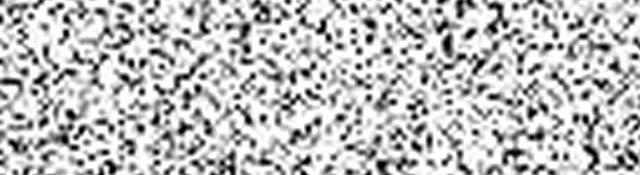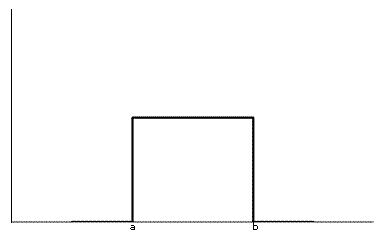## Uniform Noise

Uniform noise means the different "values" of noise are equally probably. Its probability density function looks something like this:If you don't understand what this graph (probability distribution function) means, you might want to have a look at the article on Noise Models.

## Generating uniform random numbers

Here the plan of action to generate uniform noise: For each pixel in the image, we generate a random number that follows the distribution shown above. Then we simply add the random number to the pixel's original value. Basically thats what noise is. Corrupting an image.

By default, the random numbers generated by computers are uniform in nature. So we won't need to put in any effort to generate the random numbers (normal C/C++ functions will do). Its going to be really simple.

## The project

Begin by creating a Win32 console new project. Name it whatever you want and just accept the default settings. You'll get an empty project.

In the main cpp file, add the following headers... we'll be using them:

```#include <cv.h>
#include <highgui.h>
#include <math.h>
#include <float.h>
```

We also need to include the OpenCV libraries. Goto Project > Properties > Configuration > Linker > Input and type in cv.lib cxcore.lib highgui.lib cvaux.lib in Additional Dependencies.

Now onto the code. We'll first add code that generates uniformly distributed random numbers. Since C/C++ functions themselves generate uniform random numbers, we just generate a random number, and simply return it.

```// Returns a uniformly distributed random number
double uniform()
{
return (rand()/(float)0x7fff)-0.5;
}
```

rand is a standard function that returns a random number betwen 0 and 32767 (0x7FFF). So we convert it into a decimal number between 0.0 and 1.0 and then we convert it into a numebr between -0.5 and +0.5, then we return it.

Now for the actual generation of noise. We start off by writing a function that returns the noisy image:

```IplImage* GenerateNoise(IplImage* img, float amount=255)
{
```

We create a copy of the image, just to keep the original safe:

```    CvSize imgSize = cvGetSize(img);
IplImage* imgTemp = cvCloneImage(img); // This will hold the noisy image
```

And we go through each pixel of the image:

```    // Go through each pixel
for(int y=0;y<imgSize.height;y++)
{
for(int x=0;x<imgSize.width;x++)
{
```

Now we generate a random number that will be the amount of noise added (or subtracted) to the pixel's current value:

```            int randomValue = (char)((uniform())*amount);
```

The uniform() function returns a uniformly distributed random number between -0.5 and 0.5 This gets multiplied with the amount (rather, magnitude) of noise you want.

With the number in hand, we "add' this nosie to the current pixel:

```            // Here we "apply" the noise to the current pixel

int pixelValue = cvGetReal2D(imgTemp, y, x)+randomValue;

// And set this value in our noisy image
cvSetReal2D(imgTemp, y, x, pixelValue);
```

And with the we close both loops, and return the image we've created.

```        }
}
// return
return imgTemp;
}
```

Simple enough, right?

## Applying noise

Now lets get to the main() function:

```int main()
{
// Load the image with no noise
IplImage* imgTemp;
```

We simply load an image, img. We'll be adding noise to this image, which will be stored in imgTemp. Now the img is loaded as a grayscale image.

Next we create a window that will display the noisy image. We'll also be adding a trackbar so you can experiment with the "amount" parameter.

```    // Initialize the window systems
int trackPos = 20;
cvNamedWindow("Image");
cvCreateTrackbar("amount", "Image", &trackPos, 255, NULL);
```

This creates a window named "Image", and adds a trackbar named "amount" to it. If you're not sure about trackbars, you might want to go through the article HighGUI: Creating Interfaces.

Next we create a loop that runs till infinity. And inside this loop, we apply noise to image we just loaded, then we display it, and we immediately release it (to avoid enormous memory usage):

```    while(true)
{

imgTemp = GenerateNoise(img, trackPos);

// Display it all
cvShowImage("Image", imgTemp);
cvReleaseImage(&imgTemp);
```

We then check for a keypress. If a key is pressed, we need to break out of the loop:

```        // Check for a key press
char keyPress = cvWaitKey(10);
if(keyPress!=-1) break;
}
```

And once we're out of the loop, we release the original image, and, well, exit.

```    // Finally, exit
cvReleaseImage(&img);
return 0;
}
```

And thats all there is to generating uniform noise!

## Wrap up

Here you learned how to create uniform noise and we create a little interactive demo out of it, where noise is applied to the image in real time and you can alter the amount of noise.

We limited our noise to a grayscale image. To extend this to a coloured image, you take the three channels, and apply noise to each of them independently. And voila, you get a noisy coloured image!

There also exist other types of noises, exponential, rayleigh. You can read up about them here: Noise models.

#### Related posts#### 7 unique neural network architectures